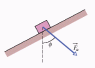# Work problem, box moves at h=.25 with F=85N

• edgarpokemon
The box moves up a frictionless ramp at a constant speed. Fa is the force that is applied to the box and it causes it to move. The box moves up a frictionless ramp at a constant speed and the box uses Fa to move. The box moves up a frictionless ramp at a constant speed and the box uses Fa to move.

## Homework Statement

A constant force Fa of magnitude 85.0N is applied to a 3.0kg shoe box at angle 55.0, causing the box to move up a frictionless ramp at constant speed. How much work is done on the box by Fa when the box has moved through vertical distance h=.25m?

## Homework Equations

mgsin(angle)-Fa=0(constant speed)
mgsin(55)=Fa=24.08N
hyp=opp/sin(55)=.305m
Work=+7.35J (problably minus because the work is pointing down and displacement is up)
mgh=-7.35J

## The Attempt at a Solution

So I know that the simple way is just to use mgh. But in my homework the the box is going up, so I assume that the gravity will do negative work. But the answer is positive and they used mgh. They used Wa=-Wg and they they switched the -Wg to +Wg, and i am not sure why they did that. So was what I did correct too?

#### Attachments

•mmm.gif
2.5 KB · Views: 431
Hello Ed,

I don't see ##m\vec g\sin\theta## and ##\vec F_a## pointing in the same direction ? I agree that the component of ##\vec F_a## along the incline should be 24.1 Newton (my ##|\vec g| = 9.81## m/s2). And if ##\vec F_a## does no work perpendicular to the incline (there is no displacement in that direction), but only along the incline, over the given distance, I get a different result !

 I suspect this exercise is a lot more involved then you (and I at first) think. Where in the drawing is the ##55 \ ^\circ## ? Is ##\phi = 55^\circ##. In that case: check: "frictionless" and "at constant speed" !
[edit2] suspicion relieved, see below. And I don't get a different answer any more . But ##Fa## is an unknown.

Last edited:
BvU said:
 I suspect this exercise is a lot more involved then you (and I at first) think.
I would suggest it is rather less involved than it appears. The trick is to realize what information is irrelevant.
Ed, think of it from the perspective of the box and its overall change of state.

Frictionless and the angle of the incline is not given !

BvU said:
Frictionless and the angle of the incline is not given !
Is that in response to my post? If so, what point are you making?